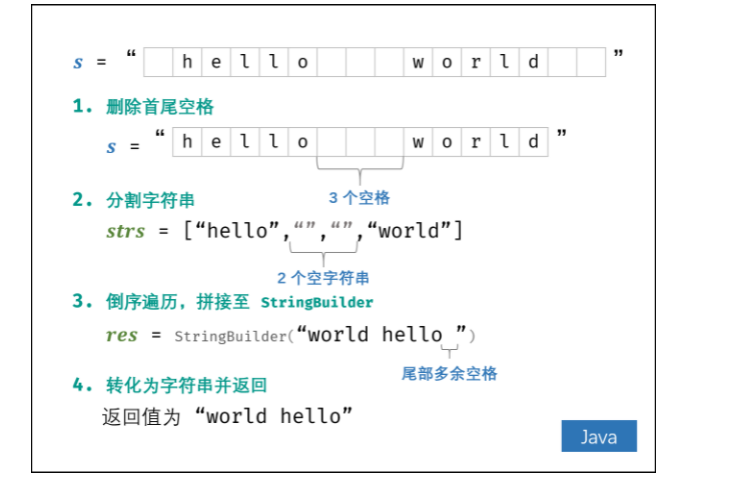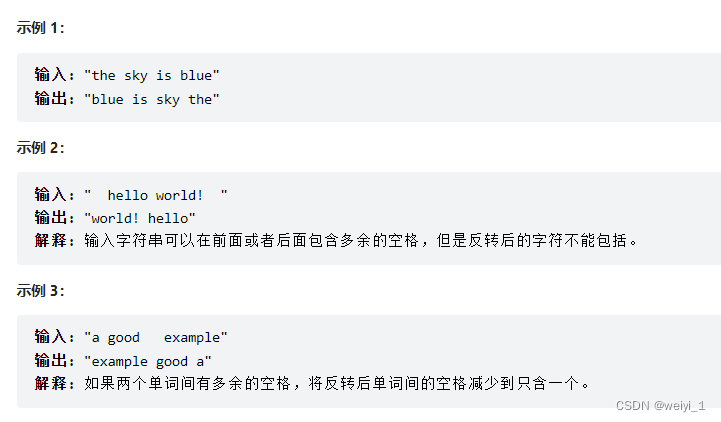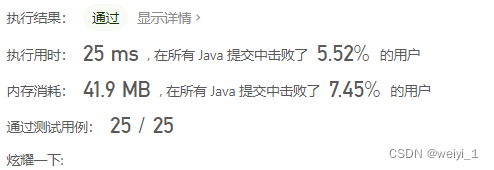• 翻转单词顺序
2021-05-24 09:07:01

题目：翻转单词顺序

参考文献：

剑指offer 何海涛老师 P220

1.翻转整个句子

如 I  am alex! ---> !xela am I

void reverseSentence(char *sen,int n){

int start=0;

//!int end1 = sizeof(sen)/sizeof(sen)-1;

// !printf("end1=%d\n",end1);

int end = n-1;

//printf("end=%d\n",end);

//不能用start<=n/2。

while(start

//A^0 = A; A^1 = A的各位相反

//A^A=0 A=A^B B=B^A=B^A^B=A; A=A^B=A^B^A=B

sen[start] ^= sen[end];

sen[end] ^= sen[start];

sen[start] ^= sen[end];

start++;

end--;

}

}

2.翻转单词顺序

如I am alex! --> alex! am I 。其实只需要在1的基础上继续将每个单词顺序颠倒。

void reverseWordsInSentence(char *sen){

char *high,*low;

reverseSentence(sen,strlen(sen));

printf("sen转换后：\n");

puts(sen);

high = sen;

low = sen;

printf("word转换后：\n");

while(*high!='\0'){

//找到不是空格的字符即为单词的头。

while(*high==' ' && *high!='\0'){

high++;

}

low = high;

//找到空格的字符即为单词结尾的下一个字符

while(*high!=' ' && *high!='\0'){

high++;

}

//此时交换一个单词的首尾

reverseSentence(low,high-low);

puts(sen);

}

}

3.左旋转字符串。

如abceefg 左旋转2个字符得到ceefgab

void leftReverse(char *sen,int n){

char *high;

int len = n, i=0;

high = sen;

//添加边界值判断。当n==strlen(sen)不需要旋转 。当n<0也不需要旋转。当字符串为空，更不用旋转

if(strlen(sen) >0 && n>0 && n < strlen(sen)){

for(;i

high++;

}

reverseSentence(sen,len);

printf("%c,%d\n",*high,strlen(sen)-len);

reverseSentence(high,strlen(sen)-len);

reverseSentence(sen,strlen(sen));

}

}

下面给出c++实现的版本：

c++是string 操作有一些方法，如求长度 str.length()，对字符串的修改不会反映到字符串中，要保存结果可以用replace() 替换字符。

如string s = "abcdefg";s.replace(2,3,"!!!!!");//从索引2开始3个字节的字符全替换成"!!!!!"

string ReverseSentence(string str) {

int high,low;

str=reverseSentence(str,str.length());

cout<

high = 0;

low = 0;

string temp;

while(str[high]!='\0'){

//找到不是空格的字符即为单词的头。

while(str[high]==' ' && str[high]!='\0'){

high++;

}

low = high;

//找到空格的字符即为单词结尾的下一个字符

while(str[high]!=' ' && str[high]!='\0'){

high++;

}

//此时交换一个单词的首尾

temp=reverseSentence(str.substr(low,str.length()),high-low);

cout<

str.replace(low,str.length(),temp);

}

return str;

}

string reverseSentence(string sen,int n){

int start=0;

//!int end1 = sizeof(sen)/sizeof(sen)-1;

// !printf("end1=%d\n",end1);

int end = n-1;

//printf("end=%d\n",end);

//不能用start<=n/2。

while(start

//A^0 = A; A^1 = A的各位相反

//A^A=0 A=A^B B=B^A=B^A^B=A; A=A^B=A^B^A=B

sen[start] ^= sen[end];

sen[end] ^= sen[start];

sen[start] ^= sen[end];

start++;

end--;

}

return sen;

}

string leftReverse(string str, int n) {

int high;

int len = str.length(), i=0;

high = 0;

string temp,temp2;

//添加边界值判断。当n==strlen(sen)不需要旋转 。当n<0也不需要旋转。当字符串为空，更不用旋转

if(len>0 && n>0 && n < len){

for(;i

high++;

}

temp=reverseSentence(str,n);

cout<

str.replace(0,temp.length(),temp);

temp2=reverseSentence(str.substr(high,len),len-n);

str.replace(high,len,temp2);

str=reverseSentence(str,len);

}

return str;

}

更多相关内容
• 给大家汇总介绍了C++实现翻转单词顺序的三种方法，都非常的简单,需要的朋友可以参考下
• 剑指offer 58-Ⅰ翻转单词顺序 class Solution { public String reverseWords(String s) { s = s.trim(); int j = s.length() - 1, i = j; StringBuilder sb = new StringBuilder(); while(i >= 0){ while...

剑指offer 58-Ⅰ翻转单词顺序class Solution {
public String reverseWords(String s) {
s = s.trim();
int j = s.length() - 1, i = j;
StringBuilder sb = new StringBuilder();
while(i >= 0){
while(i >= 0 && s.charAt(i) != ' '){
i--;
}
sb.append(s.substring(i + 1, j + 1) + " ");
while(i >= 0 && s.charAt(i) == ' '){
i--;
}
j = i;
}
return sb.toString().trim();
}
}


展开全文java leetcode
• 输入一个英文句子，翻转句子中单词顺序，但单词内字符的顺序不变。 为简单起见，标点符号和普通字母一样处理。 例如输入字符串"I am a student. “，则输出"student. a am I”。 示例 1： 输入: “the sky is ...

## 任务概述

输入一个英文句子，翻转句子中单词的顺序，但单词内字符的顺序不变。
为简单起见，标点符号和普通字母一样处理。
例如输入字符串"I am a student. “，则输出"student. a am I”。

示例 1：

输入: “the sky is blue”
输出: “blue is sky the”

示例 2：

输入: " hello world! "
输出: “world! hello”
解释: 输入字符串可以在前面或者后面包含多余的空格，但是反转后的字符不能包括。

示例 3：

输入: “a good example”
输出: “example good a”
解释: 如果两个单词间有多余的空格，将反转后单词间的空格减少到只含一个。

说明：

无空格字符构成一个单词。
输入字符串可以在前面或者后面包含多余的空格，但是反转后的字符不能包括。
如果两个单词间有多余的空格，将反转后单词间的空格减少到只含一个。

## 解题思路

思路1：

1. 如果输入是："    the sky is blue   "
2. 前面和后面都有空格，这时要用 trim() 方法-----------用于删除字符串的头尾空白符。
3. 得到”the sky is blue“这个首尾都没空格的字符串
4. 然后定义两个变量 i 和 j ，j 不动，i 从最后开始向前面扫描
5. 扫描的时候进行判断，如果i>=0 && s.charAt(i)!=’  ’ 那么 i 就自减一 ，继续向前扫描
charAt() 方法---------------用于返回指定索引处的字符。索引范围为从 0 到 length() - 1,包含头不包含尾。
6. 将扫描的单词进行添加到一个新的字符串StringBuilder中
当对字符串进行修改的时候，需要使用 StringBuffer 和 StringBuilder 类。 和 String 类不同的是，StringBuffer 和 StringBuilder 类的对象能够被多次的修改，并且不产生新的未使用对象。
7. 扫描完第一个单词之后，开始扫描单词之前的空格
8. 进行判断 如果i>=0&&s.charAt(i)==’  ’ 跳过单词间的空格
9. 完了之后把 j 的下标传到下一个单词的尾端，开始新的一轮的扫描。

代码如下

class Solution {
public String reverseWords(String s) {
s=s.trim();//删除首尾的空格
int j=s.length()-1;
int i=j;
StringBuilder res=new StringBuilder();
while(i>=0){
while(i>=0&&s.charAt(i)!=' ')i--;//搜素首个空格
res.append(s.substring(i+1,j+1)+" ");//添加单词
while(i>=0&&s.charAt(i)==' ')i--;//跳过单词间的空格
j=i;//J指向下一个单词的尾字符
}
return res.toString().trim();//转为字符串并返回

}
}


思路2代码如下

class Solution {
public String reverseWords(String s) {
String[] strs = s.trim().split(" "); // 删除首尾空格，分割字符串
StringBuilder res = new StringBuilder();
for(int i = strs.length - 1; i >= 0; i--) { // 倒序遍历单词列表
if(strs[i].equals("")) continue; // 遇到空单词则跳过
res.append(strs[i] + " "); // 将单词拼接至 StringBuilder
}
return res.toString().trim(); // 转化为字符串，删除尾部空格，并返回
}
}


## 总结

上面提供了两种方法，其实质都是先去除首尾的空格，然后对处理过的字符串进行不同的处理方法，不管是从后面往前面扫描还是倒叙遍历一个数组，都可以达到效果。

展开全文字符串 java 算法
• 主要为大家详细介绍了java实现翻转单词顺序列，具有一定的参考价值，感兴趣的小伙伴们可以参考一下
• 输入一个英文句子, 翻转句子中单词顺序, 但单词内字符的顺序不变. 为简单起见, 标点符号和普通字母一样处理. 例如输入字符串"I am a student.", 则输出"student. a am I".或“student. a am I”翻转成“I am a ...
•leetcode
• 输入一个英文句子，翻转句子中单词顺序，但单词内字符的顺序不变。为简单起见，标点符号和普通字母一样处理。例如输入字符串"I am a student. “，则输出"student. a am I”。 示例： 思路： 定义两个字符串，第...

## 题目描述：

输入一个英文句子，翻转句子中单词的顺序，但单词内字符的顺序不变。为简单起见，标点符号和普通字母一样处理。例如输入字符串"I am a student. “，则输出"student. a am I”。

## 示例：## 思路：

定义两个字符串，第一个用来储存返回对象，第一个动态存储单词。遍历字符串。时间复杂度为O（n）

## 代码：

class Solution {
public String reverseWords(String s) {
if(s.length()==0){
return "";
}
if(s.length()==1){
if(s.charAt(0)==' '){
return "";
}
return s;
}
//先定义一个空的字符串
String ss = "";
String a = "";
for(int i = 0;i<s.length();i++){
if(s.charAt(i)==' '){
if(ss==""){
ss = a;
a = "";
}else{
if(a != ""){
ss = a + " " + ss;
a = "";
}
}
}else{
a+=s.charAt(i);
}
}
if(s.charAt(s.length()-1) != ' '){
if(ss==""){
ss = a;
a = "";
}else{
if(a != ""){
ss = a + " " + ss;
a = "";
}
}
}
return ss;
}
}


## 结果：

把所有的特例都排除了。。。。这真的是。。。展开全文leetcode 动态规划 算法
• 本文实例为大家分享了java实现翻转单词顺序列的具体代码，供大家参考，具体内容如下最近来了一个新员工Fish，每天早晨总是会拿着一本英文杂志，写些句子在本子上。同事Cat对Fish写的内容颇感兴趣，有一天他向Fish借...
• 栈+双指针解决剑指Offer58-Ⅰ—翻转单词顺序leetcode 算法 c++ 数据结构
• 翻转单词顺序 输入一个英文句子，翻转句子中单词的顺序，但单词内字符的顺序不变。为简单起见，标点符号和普通字母一样处理。例如输入字符串"I am a student. “，则输出"student. a am I”。 思路： 用一个额外...算法
• 输入一个英文句子，翻转句子中单词顺序，但单词内字符的顺序不变。为简单起见，标点符号和普通字母一样处理。例如输入字符串"I am a student. "，则输出"student. a am I"。leetcode 算法 数据结构
•算法 leetcode 字符串
• 输入一个英文句子，翻转句子中单词顺序，但单词内字符的顺序不变。为简单起见，标点符号和普通字母一样处理。例如输入字符串"I am a student. "，则输出"student. a am I"。 示例 1： 输入: "the sky is blue" ...java 开发语言 leetcode 字符串
• 每确定一个单词的边界，则将其添加至单词列表 res ； 最终，将单词列表拼接为字符串，并返回即可。 class Solution { public String reverseWords(String s) { s = s.trim(); // 删除首尾空格 int right = s.
• 7-5 翻转单词顺序 (10 分) 给定一个字符串，翻转该字符串，翻转时单词中的字符顺序不变。例如，如果字符串为"Hello World"，则翻转后为"World Hello"。单词间以一个或多个空格分隔。注意，字符串开头和结尾都可能有...
• 因为单词需要按空格划分，利用字符串的库函数进行划分，再将获取到的字符串数组倒序遍历组成新的字符串即可。 public String reverseWords(String s) { String[] words = s.trim().split(" "); StringBuilder ...
• 输入一个英文句子，翻转句子中单词顺序，但单词内字符的顺序不变。为简单起见，标点符号和普通字母一样处理。例如输入字符串"I am a student. "，则输出"student. a am I"。 说明： 无空格字符构成一个单词。 ...java leetcode
• 给定一个字符串，翻转该字符串，翻转单词中的字符顺序不变。例如，如果字符串为"Hello World"，则翻转后为"World Hello"。单词间以一个或多个空格分隔。注意，字符串开头和结尾都可能有多个空格。输出时去掉多余的...c语言...